Next: Resistance and Resistivity Up: Electric Current Previous: Electric Circuits

## Ohm's Law

Consider, again, a simple circuit in which a steady currentflows through a single conducting wire connecting the positive and negative terminals of a battery of voltage. What is the relationship between the currentflowing in the wire and the potential differenceapplied across the two ends of the wire by the battery? If we were to investigate this relationship experimentally we would quickly conclude that the currentis directly proportional to the potential difference. In other words,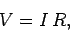(126)

where the constant of proportionalityis termed the (electrical) resistance of the wire. The above formula is called Ohm's law after its discoverer, the early nineteenth century German physicist Georg Simon Ohm. The unit of electrical resistance is the ohm (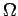), which is equivalent to a volt per ampere: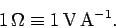(127)

There is a slight discrepancy between what we are saying now, and what we said earlier. In Sect. 5, we maintained that the electric field inside a conductor is zero. However, if there is a potential differencebetween the beginning and the end of a conducting wire, as described above, then there must be an electric field running along the length of the wire. In fact, if the wire is straight, and the electric potential decreases uniformly with distance traveled along the wire, then the longitudinal electric field-strength is given by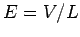(see Sect. 5.3), where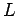is the length of the wire. The earlier result that there is zero electric field inside a conductor is equivalent to saying that conductors possess zero electrical resistance. This follows because ifis zero then the electric field, and, hence, the potential difference, must be zero, otherwise an infinite current would flow according to Ohm's law. It turns out that good conductors (i.e., copper, silver, aluminium, and most other metals) possess non-zero electrical resistances. However, these resistances are generally so small that if we were to connect the terminals of a battery together using a wire fashioned out of a good conductor then the current which would flow in the wire, according to Ohm's law, would be so large that it would damage both the wire and the battery. We usually call such a circuit a short-circuit. In order to prevent excessively large currents from flowing, conventional electric circuits contain components, called resistors, whose electrical resistance is many orders of magnitude greater than that of the conducting wires in the circuit. When we apply Ohm's law,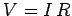, to a circuit, we usually only count the net resistanceof all the resistors in the circuit, and neglect the resistances of the interconnecting wires. This means that all of the major drops in electric potential, as we travel around the circuit from one terminal of the battery to the other, take place inside the resistors. The drop in potential in the conducting wires themselves is usually negligible. Thus, to all intents and purposes, good conductors, and wires made out of good conductors, act as if they have zero resistance, and contain zero electric field.Next: Resistance and Resistivity Up: Electric Current Previous: Electric Circuits
Richard Fitzpatrick 2007-07-14